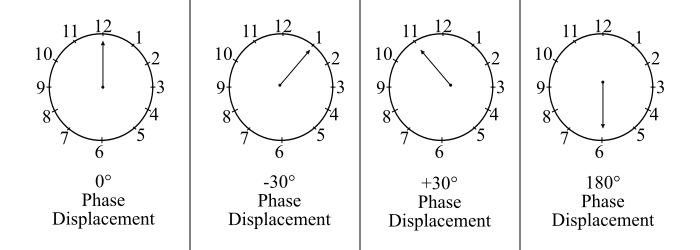# Three-Phase Transformer Vector Groups – How are the Vector Groups Divided?

The three-phase transformer vector groups are divided into four main groups depending on the phase difference between the corresponding line voltages on the high-voltage and lowvoltage sides. The angle by which the line voltage on the low-voltage side lags behind the line voltage on the high-voltage side is called as the phase difference and it is measured in the units of 30° in the clockwise direction.

The four transformer vector groups are −

• Group 1 – No Phase Displacement, i.e., 0° Phase Displacement

• Group 2 – Phase Displacement of 180°

• Group 3 – Phase Displacement of -30°

• Group 4 – Phase Displacement of +30°

Now, instead of expressing the phase displacement between the line voltages in degrees, it is more convenient to use clock method of angle designation. In the clock method, the phasor of the line voltage on the high-voltage side is considered as the minute hand of the clock and fixed at 12 O’clock.

The phasor of the line voltage on the low-voltage side is considered as the hour hand and set on the dial of the clock depending upon the phase displacement with respect to the line voltage on high-voltage side. Since, the angle of 30° is the angle between the two adjacent figures on the dial of the clock. Hence, it can be taken as the unit of dial shift. Therefore, using the clock method, the phase displacement between the line voltage on high-voltage side and the line voltage on low-voltage side can be given as follows −## Case 1

When the hour hand of the clock is at 12 O’ clock position, then the phase displacement is zero i.e. the connection of the transformer is of the group 1.

## Case 2

When the hour hand of the clock is at 6 O’ clock position, the phase difference is (6×30°) = 180°. Thus, the transformer connection is of the group 2.

## Case 3

When the hour hand of the clock at 1 O’ clock position, then the phase difference is -30° (in clockwise direction) and the transformer connection is of the group 3.

## Case 4

When the hour hand of the clock at 11 O’ clock position, then the phase difference is +30° (in anti-clockwise direction) and the transformer connection is of the group 4.

Therefore, the numbers 0 (or 12), 6, 1 and 11 are the group reference numbers and indicates the phase shift between the low-voltage side line voltage and high-voltage side line voltage.

For example, a transformer connection Yd11 gives the following information −

• Y (upper case letter shows low-voltage winding) indicates that the high voltage windings are star connected.

• d (lower case letter shows low-voltage winding) indicates that the low-voltage winding is delta connected.

• 11 shows that the line voltage on low-voltage side lags the line voltage on the highvoltage side by (11×30°) = 330°, measured from high-voltage phasor in the clockwise direction. In other words, the line voltage on low-voltage side leads the line voltage on the high-voltage side by +30°, measured from high-voltage phasor in the anticlockwise direction.

Important – The transformers in the same vector groups can only be connected in parallel.